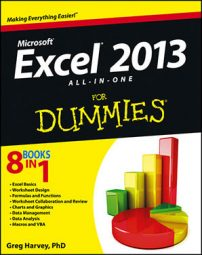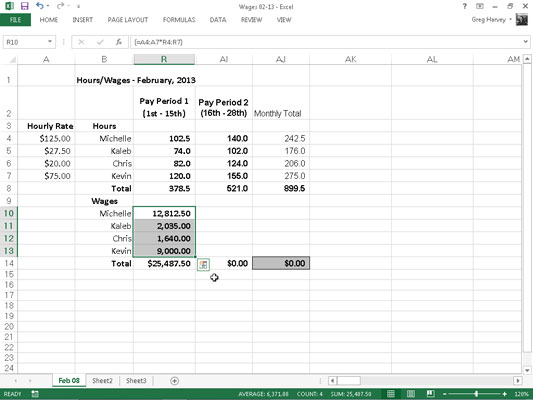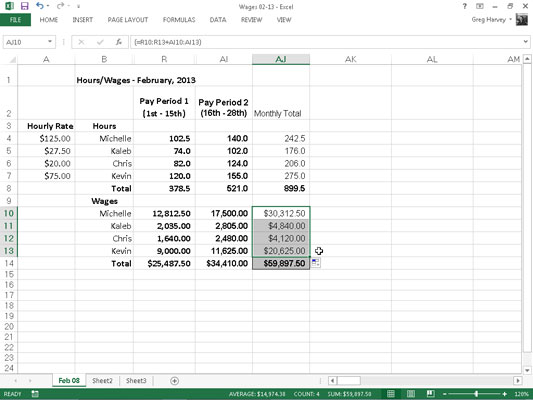##### Excel 2013 All-in-One For DummiesTo get an idea of how you build and use array formulas in an Excel 2013 worksheet, consider the sample worksheet. This worksheet is designed to compute the biweekly wages for each employee. It will do this by multiplying each employee’s hourly rate by the number of hours worked in each pay period. Instead of creating the following formula in cell R10, you copy down the cells R11 through R13:`=A4*R4`

You can create the following array formula in the array range:

`={A4:A7*R4:R7}`

This array formula multiplies each of the hourly rates in the 4 x 1 array in the range A4:A7 with each of the hours worked in the 4 x 1 array in the range R4:R7. This same formula is entered into all cells of the array range (R10:R13) as soon as you complete the formula in the active cell R10.

To see how this is done, follow along with the steps required to build this array formula:

1. Make cell R10 the current cell, and then select the array range R10:R13 and type = (equal sign) to start the array formula.

You always start an array formula by selecting the cell or cell range where the results are to appear. Note that array formulas, like standard formulas, begin with the equal sign.

2. Select the range A4:A7 that contains the hourly rate for each employee as shown, type an * (asterisk for multiplication), and then select the range R4:R7 that contains the total number of hours worked during the first pay period.

3. Press Ctrl+Shift+Enter to insert an array formula in the array range.

When you press Ctrl+Shift+Enter to complete the formula, Excel inserts braces around the formula and copies the array formula {=A4:A7*R4:R7} into each of the cells in the array range R10:R13.

When entering an array formula, you must remember to press Ctrl+Shift+Enter instead of just the Enter key because this special key combination tells Excel that you are building an array formula, so that the program encloses the formula in braces and copies it to every cell in the array range.

Also, don’t try to create an array formula by editing it on the Formula bar and then insert curly braces because this doesn’t cut it. The only way to create an array formula is by pressing Ctrl+Shift+Enter to complete the formula entry.

Following is the February wage table after completing all the array formulas in three ranges: R10:R13, AI10:AI13, and AJ10:AJ13. In the second cell range, AI10:AI13, the following array formula was entered to calculate the hourly wages for the second pay period in February:`{=A4:A7*AI4:AI7}`

In the third cell range, AJ10:AJ13, I entered the following array formula to calculate the total wages paid to each employee in February 2003:

`{=R10:R13+AI10:AI13}`

When you enter an array formula, the formula should produce an array with the same dimensions as the array range that you selected. If the resulting array returned by the formula is smaller than the array range, Excel expands the resulting array to fill the range. If the resulting array is larger than the array range, Excel doesn’t display all the results.

When expanding the results in an array range, Excel considers the dimensions of all the arrays used in the arguments of the operation. Each argument must have the same number of rows as the array with the most rows and the same number of columns as the array with the most columns.